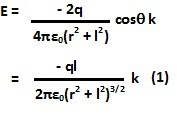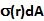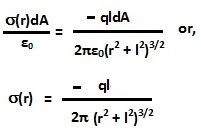## Tuesday, October 26, 2010

### Irodov Problem 3.59As described in problems 3.54 by using the method of images, we can place a negative charge -q at a distance 2l from the charge q on the other side of the conducting plane.

Consider a point on the conducting plane that is at a distance r from the perpendicular on the plane from q. The net electric field at this location will be given by,There can never be an electric field inside the body of conductor, in other words there will be no electric field just beneath the surface of the conductor. This is because, the entire conductor has to be at the same electric potential. If there were a electric field within the conductor it would mean that there would be a potential difference between two points within the conductor. Let us assume that the charge density on the conductor at a distance r from the point where the perpendicular from the charge q touches the conducting plane is.

Consider an infinitesimally small cylinderical Gaussian Pillbox, of area dA and height dh (considered to be as thin as the thickness of a plane), a this location as shown in the figure below. One flat end surface of this cylinder lies within the thickness of the conductor (it just penetrates the conductor) and other flat end is just above the surface of the conductor.
The electric field E impinges perpendicularly into the Gaussian pillbox's surface into the upper plane of the cylinder as shown. The area vector of the upper surface of the cylinder is given by (dA k). There is no electric flux through the other end since there is no electric field on that other surface (that lies just below the surface). Hence,The total charge contained within the Gaussian pillbox comes from the surface charge density of the conductor and given by. Now applying Gauss's law we have,